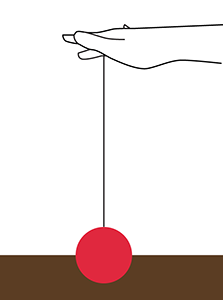# There's Something Odd About This Yo-YoYou take a yo-yo and put it on a table, like so:

The yo-yo is placed vertically, such that it can roll back and forth on the table. You hold the string and pull lightly; the string makes some angle with the vertical.

And you notice something quite strange. If you pull the string (softly) at certain angles, the yoyo rolls clockwise; at other angles, it rolls counterclockwise! The only thing that's changing is the angle $\theta$ at which you pull the yoyo string (with respect to the vertical). Try this out if you've never seen it, it's pretty awesome.

So now the question is, at what angle does the yo-yo switch its rolling from clockwise to counterclockwise, or vice-versa? That is, what is the critical angle at which the yo-yo doesn't rotate at all?

Let me give you some numbers, because Brilliant doesn't allow answers with variables. The radius of the yo-yo is $R=0.5m$, and the smaller radius of the inside spool of string is $r=0.25m$. What is the angle $\theta$ with respect to the vertical? Give your answer in degrees.

×

Problem Loading...

Note Loading...

Set Loading...